# Team for Advanced Flow Simulation and Modeling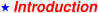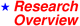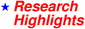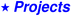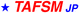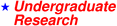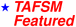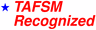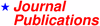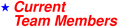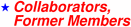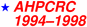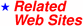tezduyar@gmail.com

## Flow Between Two Concentric Cylinders (Taylor-Couette Flow)

The purpose of this set of computations is to simulate the instabilities that develop between two concentric cylinders in relative rotation. The Reynolds number is based on the gap between the two cylinders and the speed of the inner cylinder. The outer cylinder is at rest. Beyond the critical Reynolds number, the regular Couette flow becomes unstable and one sees the development of Taylor vortices. Further increases in the Reynolds number leads to an unsteady flow pattern.

The finite element mesh employed consists of 38,400 hexahedral elements and 45,024 nodes. The mesh contains 6 elements in the radial direction, 32 elements in the circumferential direction, and 200 elements in the axial direction. At every time step, a set of 282,366 nonlinear equations resulting from the finite element discretization are solved iteratively. As boundary conditions, at the upper and lower boundaries, the axial component of the velocity and the x and y components of the stress vector are set to zero (the z-axis lies in the axial direction).

In the first case considered, the Reynolds number is 150. This is greater than the critical Reynolds number, and therefore, for certain disturbances, one would expect the Couette flow to develop instabilities. When the solution is computed with no external disturbances, a stable Couette flow is observed. On the other hand, if the solution is obtained with an initial condition that corresponds to an unsteady solution from a higher Reynolds number, a Taylor vortex is realized. The figure below shows the axial velocity at the vertical, cylindrical, and horizontal sections. The cylindrical section lies midway between the inner and outer surfaces. We observe that the solution at this Reynolds number is axisymmetric.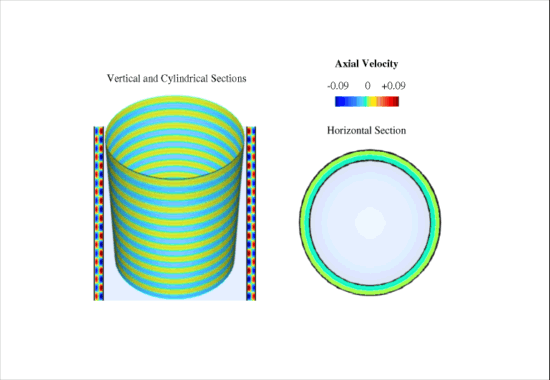At Reynolds number 250 our computations reveal the presence of wavy vortex flow. At this Reynolds number the solution is no longer axisymmetric and involves 3D instabilities. The figure below shows the axial velocity at the vertical, cylindrical, and horizontal sections for a nearly temporally periodic solution. We observe that in addition to the cells in the axial direction, there are four waves traveling in the azimuthal direction. The mesh generator, flow solver, and flow visualization software (based on Wavefront) were developed by the T*AFSM.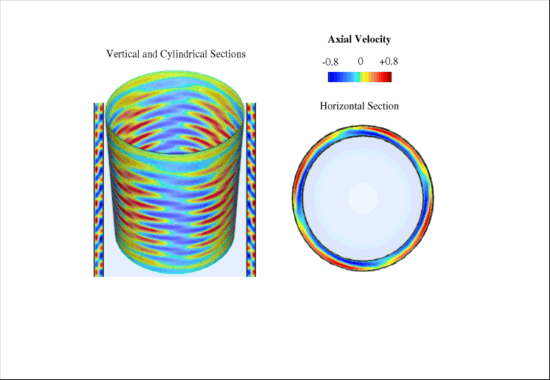### References:

1. T.J.R. Hughes, T.E. Tezduyar and A.N. Brooks, "Streamline Upwind Formulations for Advection-Diffusion, Navier-Stokes, and First-order Hyperbolic Equations", Proceedings of the Fourth International Conference on Finite Element Methods in Fluid Flow, University of Tokyo Press, Tokyo (1982).

2. T.E. Tezduyar, "Stabilized Finite Element Formulations for Incompressible Flow Computations", Advances in Applied Mechanics, 28 (1991) 1-44.

3. T.E. Tezduyar, S. Mittal and R. Shih, "Time-accurate Incompressible Flow Computations with Quadrilateral Velocity-Pressure Elements", Computer Methods in Applied Mechanics and Engineering, 87 (1991) 363-384.

4. T.E. Tezduyar, S. Mittal, S.E. Ray and R. Shih, "Incompressible Flow Computations with Stabilized Bilinear and Linear Equal-order-interpolation Velocity-Pressure Elements", Computer Methods in Applied Mechanics and Engineering, 95 (1992) 221-242.

5. T.E. Tezduyar, S.K. Aliabadi, M. Behr and S. Mittal, "Massively Parallel Finite Element Simulation of Compressible and Incompressible Flows", Computer Methods in Applied Mechanics and Engineering, 119 (1994) 157-177.